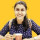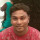1. Home >
2. Apps >
3. Groups >

# Write an efficient algorithm to find the first non-repeated character in a string defined

Question asked by Banashree Patra in #Coffee Room on Apr 23, 2012Banashree Patra · Apr 23, 2012
Rank D2 - MASTER
Write an efficient algorithm to find the first non-repeated character in a string defined
over the English alphabet set [a-z, A-Z].
For example, the first non-repeated character in teeter is r.
Analyze the time complexity of your algorithm.
NOTE:Time complexity should be linear not quadratic . Posted in: #Coffee RoomAnkita Katdare · Apr 29, 2012
Rank A1 - PRO
A simple algorithm should be:

Step 1. Scanning the string from left to right and then storing the values in a count array.
Step 2. Scanning the string from left to right again and checking for count of each character, if an element who's count is 1 is found, return it.Rank A1 - PRO
A simple algorithm should be:

Step 1. Scanning the string from left to right and then storing the values in a count array.
Step 2. Scanning the string from left to right again and checking for count of each character, if an element who's count is 1 is found, return it.
AKD, the algorithm of yours seems to be quadratic time complexity!Ankita Katdare · May 6, 2012
Rank A1 - PRO
Praveen-Kumar
AKD, the algorithm of yours seems to be quadratic time complexity!
Hmm, yes. O(n2) Praveen-Kumar write one algo here for us all.Rank A1 - PRO
Hmm, yes. O(n2) Praveen-Kumar write one algo here for us all.
Er... Will try... If PHP I guess I can easily say... There is a concept of associative arrays, where you can do this way: count["char"] = n and so on.

For the algorithm,
1. Define an empty associative array and initialize to 0. def count[] = 0;
2. For each character, check the occurrence and increment the particular array node. count["s"]++;
3. Loop through the array and get the first value, which is 1!
```foreach (val as count)
if val is 1
print the index```
The complexity is O(n).silverscorpion · May 6, 2012
Rank A3 - PRO
A simple algorithm to do it in only one pass of the string is to maintain an array of count of the letters, and then scanning through the count array instead of the string itself again.

The algorithm is like:

```initialize count to all zeros
for i in range 0 to length(S):
if count[S[i]] == 0:
count[S[i]] = i
count[S[i]]++
Index = length(S)
for i in range 1 to 255:
if count[i] == 1:
if count[i] < Index:
Index = count[i]
return S(Index)```
I hope the pseudo code is clear..

The running time is O(n+c) where c is 256 here. So, essentially it is O(n)silverscorpion · May 6, 2012
Rank A3 - PRO
Praveen-Kumar
Er... Will try... If PHP I guess I can easily say... There is a concept of associative arrays, where you can do this way: count["char"] = n and so on.

For the algorithm,
1. Define an empty associative array and initialize to 0. def count[] = 0;
2. For each character, check the occurrence and increment the particular array node. count["s"]++;
3. Loop through the array and get the first value, which is 1!
```foreach (val as count)
if val is 1
print the index```
The complexity is O(n).
I think that won't work.. If you are using associative arrays, you need to specify the count of the character as well as the index when it was first encountered.

For example, take the string "bbzaccddbcd"
If you fill an associative array with this string, with the key as the characters, you would get

array['b']=3, array['c']=3, array['a']=1, etc.. and array['z']=1

Now you will scan through the array and the first element that has the value 1 is 'a'. So, you will return a, while the answer is z.

That's why, you also need to store the index where each character is first encountered. Then compare that value also.. Just checking for the count being 1 is not enough.Rank A1 - PRO
silverscorpion
I think that won't work.. If you are using associative arrays, you need to specify the count of the character as well as the index when it was first encountered.

For example, take the string "bbzaccddbcd"
If you fill an associative array with this string, with the key as the characters, you would get

array['b']=3, array['c']=3, array['a']=1, etc.. and array['z']=1

Now you will scan through the array and the first element that has the value 1 is 'a'. So, you will return a, while the answer is z.

That's why, you also need to store the index where each character is first encountered. Then compare that value also.. Just checking for the count being 1 is not enough.
Hey wait... Coming to what you said, consider the string "bbzaccddbcd":
```count["b"]++;
count = {
"b" = 1
}
count["b"]++;
count = {
"b" = 2
count["z"]++;
}
count = {
"b" = 2,
"z" = 1
}
...
...
...
...
count["d"]++;
count = {
"b" = 2,
"z" = 1,
"a" = 1,
"c" = 3,
"d" = 3
}```
When the loop encounters the first value of 1, it returns "z" and not "a". Hope you are clear.

## You must log-in or sign-up to reply to this post.

Click to Log-In or Sign-Up